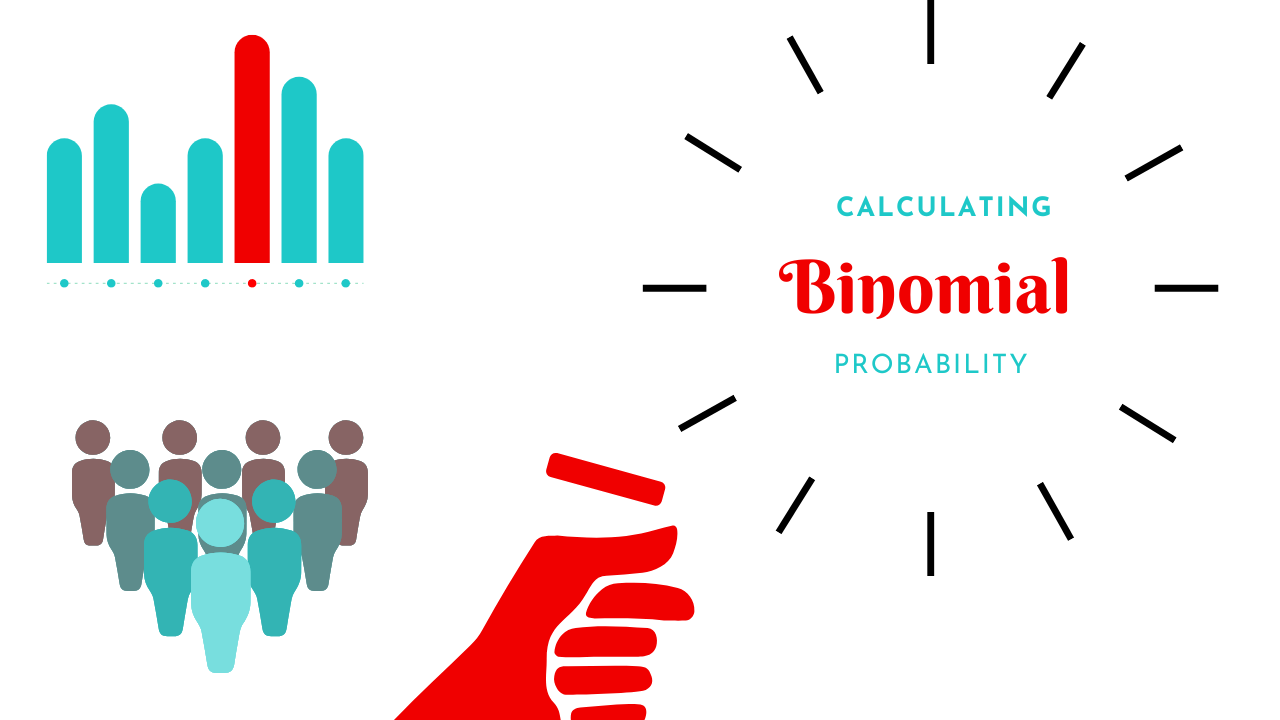# How Do I Calculate a Binomial Probability From a Forced-Choice Measure?

Apr 21, 2023

If you need more in-depth information than this article provides about the method and practice of this concept, you can find that here.

## What is a Binomial Probability?

A binomial distribution is the probably of getting one of two possible outcomes across repeated trials, when each trial has the same chance of getting one outcome. The value is essentially calculated by multiplying independent trials by successes.

So, if you flip a coin 50 times, your probability of getting heads is 50%. And 50x 0.5 = 25, which also passes the common sense test, because with a balanced coin we'd expect about half heads and half tails. So the distribution formula describes the likelihood of getting a specific number of outcomes (in our case, "correct") across an experiment with a set number of trials. This also requires that the trials are independent of each other - when you flip a coin a second time, the value from the first flip doesn't influence the second one. The probability of the flip is 50% each time.

Where the distribution formula comes in is when you want to calculate the probability of getting "heads" six times in ten tries. If that probability is about .02, then we'd say there's a 20% chance that this outcome will occur each time. Which is pretty high odds. We wouldn't suspect there's anything wrong with our coin with an outcome that should happen by chance once every five times. But if our odds show that an outcome is very, very improbable and it happens anyway, then we might become very suspicious of that coin. We might try a new set of trials or look for other data to prove that the coin is weighted.

This is exactly what we're doing when we run a binomial calculation on a forced-choice measure. We're calculating the probability of that outcome and using that information to determine whether that outcome fits random ("chance") patterns, or non-random ("below-chance") patterns that suggest our coin was weighted.

## How Do I Calculate It?

### Step 1:

Go to a probability calculator such as the one here.

### Step 2:

Input “n” (the number of questions, or trials from the measure.

Input “k” (the number answered correctly)

Input “p” which is .05. (you can leave “q”)

### Step 3: Calculate significance.

I usually use the middle number, which is the probability of getting the exact or fewer questions correct.

Your first check is to see if it’s below .05. If it is, then you have a statistically significant finding. That means that your pattern is likely not random.

### Step 4: Convert to odds.

Use your decimal columns – so let’s say your number is .00054136.

The first zero is “tens.”

The second zero is "hundreds.”

The third zero is "thousands.”

So the fourth zero is "ten thousands.”

And so on.

In this case, this means that your odds are about 5 in 10,000. In other words, there is a 5 in 10k chance of obtaining your outcome by chance. This finding is “below chance” because those odds are too low to believe that it’s plausible to have happened randomly. If you ran 10k coin flip trials with the same number you used as "n," you would only get that outcome 5 times out of 10 thousand.

If you don’t understand this well enough to take it from here, or if you want more information and examples, feel free to check out our full course on Examiner-Designed Forced Choice Measures.

Here at Streamline, we're committed to using our powers of psychology for good. Starting from the science and empirical literature but arriving at the core of what matters. Focusing on what readers and consumers truly want. Keeping sight of what clinicians need. In our blog, videos, and courses, we’re here to serve clinical and forensic assessment psychologists, especially those early-to-mid-career with an eye toward improvement, innovation, and inspiration.